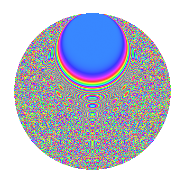# Properties

 Label 320.6.beLevel 320 Weight 6 Character orbit be Rep. character $$\chi_{320}(21,\cdot)$$ Character field $$\Q(\zeta_{16})$$ Dimension 1280 Sturm bound 288

# Related objects

## Defining parameters

 Level: $$N$$ $$=$$ $$320 = 2^{6} \cdot 5$$ Weight: $$k$$ $$=$$ $$6$$ Character orbit: $$[\chi]$$ $$=$$ 320.be (of order $$16$$ and degree $$8$$) Character conductor: $$\operatorname{cond}(\chi)$$ $$=$$ $$64$$ Character field: $$\Q(\zeta_{16})$$ Sturm bound: $$288$$

## Dimensions

The following table gives the dimensions of various subspaces of $$M_{6}(320, [\chi])$$.

Total New Old
Modular forms 1936 1280 656
Cusp forms 1904 1280 624
Eisenstein series 32 0 32

## Trace form

 $$1280q + O(q^{10})$$ $$1280q - 27184q^{22} + 44080q^{24} - 8720q^{28} - 74320q^{32} - 25520q^{34} + 139280q^{38} - 92720q^{42} - 131024q^{44} - 41760q^{51} - 146976q^{52} - 233280q^{54} - 43120q^{56} - 51984q^{58} - 57920q^{59} + 351264q^{62} - 635040q^{63} + 374880q^{64} + 254880q^{66} - 244640q^{67} + 14304q^{68} + 287680q^{71} - 828144q^{72} - 107920q^{74} - 268480q^{76} - 665328q^{78} - 355360q^{79} - 193600q^{80} + 985040q^{84} + 1443728q^{86} + 446560q^{88} - 284400q^{90} - 136880q^{94} - 2428688q^{96} - 1914608q^{98} + O(q^{100})$$

## Decomposition of $$S_{6}^{\mathrm{new}}(320, [\chi])$$ into newform subspaces

The newforms in this space have not yet been added to the LMFDB.

## Decomposition of $$S_{6}^{\mathrm{old}}(320, [\chi])$$ into lower level spaces

$$S_{6}^{\mathrm{old}}(320, [\chi]) \cong$$ $$S_{6}^{\mathrm{new}}(64, [\chi])$$$$^{\oplus 2}$$

## Hecke characteristic polynomials

There are no characteristic polynomials of Hecke operators in the database